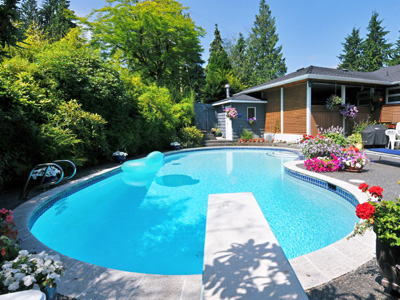Litres are the best units to measure the volume of water in a swimming pool.

# Measures (Year 5)

In KS2 Maths as children advance to Year Five their knowledge of measures will increase. By now they should be very familiar with the metric measures (millimetres, centimetres, metres, kilometres, grams, kilograms, millilitres and litres) and should be comfortable combining measures to write measurements (eg. 1.5kg = 1kg 500g) and rounding smaller measurements up or down into larger ones (eg. 740m to 1km).

Grams and kilograms are used to measure the mass or weight of things. We can write the same weight in kg (1.6kg), in g (1,600g) or by combining the two (1kg 600g). Rounding fractions of larger measures to the nearest whole number is another way of writing measurements. 1.6kg would be rounded up to 2kg. Metric and imperial measurements are used all the time in day to day life. To know your grams from your ounces and your metres from your miles will stand you in good stead, so keep practising these quizzes on measures.

How are you at combining m with km or rounding ml to the nearest l? Do you know your metric measures from your imperial ones? See how well you do in this quiz on measures.

1.
Which of these is best measured in cm?
The length of a pencil
The length of the school playground
The weight of a bag of flour
The height of a multi-storey car park
The length of the school playground and the height of a multi-storey car park would both best be measured in metres. The weight of a bag of flour should be measured in kilograms
2.
What is three quarters of 1 metre in cm?
0.75cm
75cm
750cm
7,500cm
Three quarters of 100 is 75
3.
Which unit is best to measure the volume of water in a swimming pool?
kg
ml
cm
litre
There may be 1,000s of litres of water in a swimming pool
4.
Roughly how many litres in 1 gallon?
4 litres
5 litres
8 litres
10 litres
A gallon is slightly less than 5 litres
5.
Which of these statements is true?
1 mile > 2 km
1 mile < 1.5 km
1 mile > 1.5 km
1 mile = 1.5 km
1 mile is more than 1.5 km but less than 2 km
6.
What is 156cm to the nearest metre?
1m
2m
15m
16m
As there are 100 cm in a m, this is the same as rounding to the nearest hundred
7.
How do we write 4,520ml in litres?
0.452 l
4.52 l
45.2 l
452 l
To convert millilitres to litres, divide by 1,000
8.
How many grams are equivalent to one hundredth of a kg?
0.1g
1g
1.1g
10g
There are 1,000g in a kg so 1,000 ÷ 100 = 10
9.
What is 4,321g to the nearest kg?
4kg
5kg
43kg
433kg
As there are 1,000 g in a kg, this is the same as rounding to the nearest thousand
10.
What is 6.45kg expressed in grams?
0.645g
64.5g
645g
6,450g
To convert kilograms to grams, times by 1,000